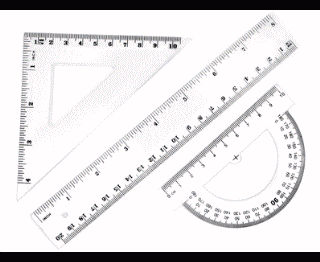Geometric Figure Processing Achievements
＜Features＞
Geometry algorithm application technology developed through map inspection system for the Geospatial Information Authority of Japan### ■2D Polygon Arithmetic

Calculating the union, intersection, and difference of polygons

### ■Intersection Calculation

Calculating the intersection between polygons, polygon and polyline, and polyline and polyline

### ■Polyline to Polygon Conversion

Extracting polygons from line drawings and enabling calculations such as area calculation and determining if a point is inside or outside the region

### ■Polygon Expansion and Contraction

Expands and contracts polygons instead of scaling them
Can expand or contract polygons to a certain distance outside or inside
Used for processes such as arranging polygons at a constant distance from each other

### ■Convex Hull

Convex hull that surrounds any given geometric object (e.g., convex hull that surrounds a text area)

### ■Scanline Conversion

Converts polygons into filled raster images
Can handle thin polygons like line drawings

### ■Topographic Map Coordinate Conversion

Converts between BL (Blue Lamp) coordinate system and UTM (Universal Transverse Mercator) coordinate system